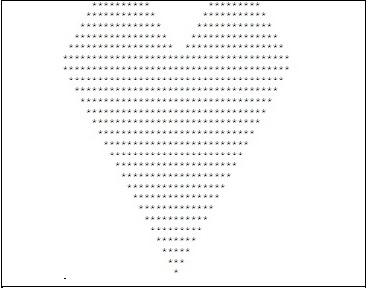# Printing Heart Pattern in C

In this program we will see how to print heart shaped pattern in C. The heart shape pattern will be look like thisNow if we analyze this pattern, we can find different section in this pattern. The base of the heart is an inverted triangle; the upper portion has two different peaks. Between these two peaks there is a gap. To make this pattern we have to manage these parts into our code to print the pattern like this.

## Example

Live Demo

#include<stdio.h>
int main() {
int a, b, line = 12;
for (a = line/2; a <= line; a = a+2) { //for the upper part of the heart
for (b = 1; b < line-a; b = b+2) //create space before the first peak
printf(" ");
for (b = 1; b <= a; b++) //print the first peak
printf("*");
for (b = 1; b <= line-a; b++) //create space before the first peak
printf(" ");
for (b = 1; b <= a-1; b++) //print the second peak
printf("*");
printf("");
}
for (a = line; a >= 0; a--) { //the base of the heart is inverted triangle
for (b = a; b < line; b++) //generate space before triangle
printf(" ");
for (b = 1; b <= ((a * 2) - 1); b++) //print the triangle
printf("*");
printf("");
}
}

## OutputUpdated on: 30-Jul-2019

6K+ Views